University Physics Volume 1

90.

(a) What is the final velocity of a car originally traveling at 50.0 km/h that accelerates opposite to the motion at a rate of $0.400m/s20.400m/s2$ for 50.0 s? Assume a coefficient of friction of 1.0. (b) What is unreasonable about the result? (c) Which premise is unreasonable, or which premises are inconsistent?

91.

A 75.0-kg woman stands on a bathroom scale in an elevator that accelerates from rest to 30.0 m/s in 2.00 s. (a) Calculate the scale reading in newtons and compare it with her weight. (The scale exerts an upward force on her equal to its reading.) (b) What is unreasonable about the result? (c) Which premise is unreasonable, or which premises are inconsistent?

92.

(a) Calculate the minimum coefficient of friction needed for a car to negotiate an unbanked 50.0 m radius curve at 30.0 m/s. (b) What is unreasonable about the result? (c) Which premises are unreasonable or inconsistent?

93.

As shown below, if $M=5.50kg,M=5.50kg,$ what is the tension in string 1?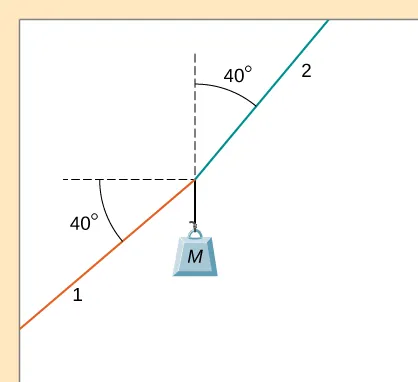94.

As shown below, if $F=60.0NF=60.0N$ and $M=4.00kg,M=4.00kg,$ what is the magnitude of the acceleration of the suspended object? All surfaces are frictionless.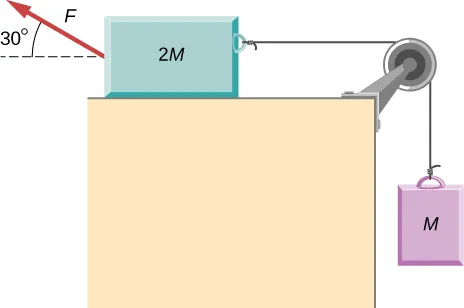95.

As shown below, if $M=6.0kg,M=6.0kg,$ what is the tension in the connecting string? The pulley and all surfaces are frictionless.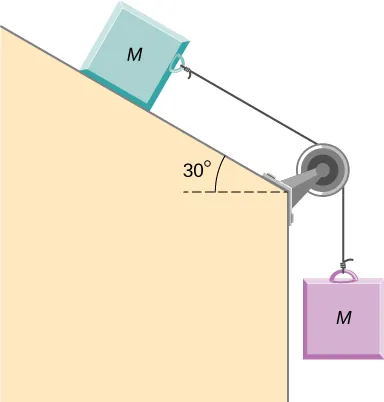96.

A small space probe is released from a spaceship. The space probe has mass 20.0 kg and contains 90.0 kg of fuel. It starts from rest in deep space, from the origin of a coordinate system based on the spaceship, and burns fuel at the rate of 3.00 kg/s. The engine provides a constant thrust of 120.0 N. (a) Write an expression for the mass of the space probe as a function of time, between 0 and 30 seconds, assuming that the engine ignites fuel beginning at $t=0.t=0.$ (b) What is the velocity after 15.0 s? (c) What is the position of the space probe after 15.0 s, with initial position at the origin? (d) Write an expression for the position as a function of time, for $t>30.0s.t>30.0s.$

97.

A half-full recycling bin has mass 3.0 kg and is pushed up a $40.0°40.0°$ incline with constant speed under the action of a 26-N force acting up and parallel to the incline. The incline has friction. What magnitude force must act up and parallel to the incline for the bin to move down the incline at constant velocity?

98.

A child has mass 6.0 kg and slides down a $35°35°$ incline with constant speed under the action of a 13.4-N force acting up and parallel to the incline. What is the coefficient of kinetic friction between the child and the surface of the incline?

99.

The two barges shown here are coupled by a cable of negligible mass. The mass of the front barge is $2.00×103kg2.00×103kg$ and the mass of the rear barge is $3.00×103kg.3.00×103kg.$ A tugboat pulls the front barge with a horizontal force of magnitude $20.0×103N,20.0×103N,$ and the frictional forces of the water on the front and rear barges are $8.00×103N8.00×103N$ and $10.0×103N,10.0×103N,$ respectively. Find the horizontal acceleration of the barges and the tension in the connecting cable.100.

If the order of the barges of the preceding exercise is reversed so that the tugboat pulls the $3.00×103-kg3.00×103-kg$ barge with a force of $20.0×103N,20.0×103N,$ what are the acceleration of the barges and the tension in the coupling cable?

101.

An object with mass m moves along the x-axis. Its position at any time is given by $x(t)=pt3+qt2x(t)=pt3+qt2$ where p and q are constants. Find the net force on this object for any time t.

102.

A helicopter with mass $2.35×104kg2.35×104kg$ has a position given by $r→(t)=(0.020t3)i^+(2.2t)j^−(0.060t2)k^.r→(t)=(0.020t3)i^+(2.2t)j^−(0.060t2)k^.$ Find the net force on the helicopter at $t=3.0s.t=3.0s.$

103.

Located at the origin, an electric car of mass m is at rest and in equilibrium. A time dependent force of $F→(t)F→(t)$ is applied at time $t=0t=0$, and its components are $Fx(t)=p+ntFx(t)=p+nt$ and $Fy(t)=qtFy(t)=qt$ where p, q, and n are constants. Find the position $r→(t)r→(t)$ and velocity $v→(t)v→(t)$ as functions of time t.

104.

A particle of mass m is located at the origin. It is at rest and in equilibrium. A time-dependent force of $F→(t)F→(t)$ is applied at time $t=0t=0$, and its components are $Fx(t)=ptFx(t)=pt$ and $Fy(t)=n+qtFy(t)=n+qt$ where p, q, and n are constants. Find the position $r→(t)r→(t)$ and velocity $v→(t)v→(t)$ as functions of time t.

105.

A 2.0-kg object has a velocity of $4.0i^m/s4.0i^m/s$ at $t=0.t=0.$ A constant resultant force of $(2.0i^+4.0j^)N(2.0i^+4.0j^)N$ then acts on the object for 3.0 s. What is the magnitude of the object’s velocity at the end of the 3.0-s interval?

106.

A 1.5-kg mass has an acceleration of $(4.0i^−3.0j^)m/s2.(4.0i^−3.0j^)m/s2.$ Only two forces act on the mass. If one of the forces is $(2.0i^−1.4j^)N,(2.0i^−1.4j^)N,$ what is the magnitude of the other force?

107.

A box is dropped onto a conveyor belt moving at 3.4 m/s. If the coefficient of friction between the box and the belt is 0.27, how long will it take before the box moves without slipping?

108.

Shown below is a 10.0-kg block being pushed by a horizontal force $F→F→$ of magnitude 200.0 N. The coefficient of kinetic friction between the two surfaces is 0.50. Find the acceleration of the block.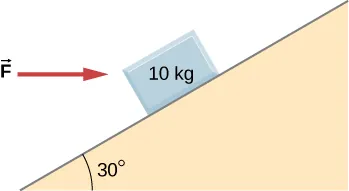109.

As shown below, the mass of block 1 is $m1=4.0kg,m1=4.0kg,$ while the mass of block 2 is $m2=8.0kg.m2=8.0kg.$ The coefficient of friction between $m1m1$ and the inclined surface is $μk=0.40.μk=0.40.$ What is the acceleration of the system?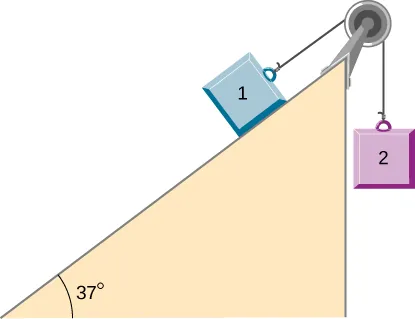110.

A student is attempting to move a 30-kg mini-fridge into her dorm room. During a moment of inattention, the mini-fridge slides down a 35 degree incline at constant speed when she applies a force of 25 N acting up and parallel to the incline. What is the coefficient of kinetic friction between the fridge and the surface of the incline?

111.

A crate of mass 100.0 kg rests on a rough surface inclined at an angle of $37.0°37.0°$ with the horizontal. A massless rope to which a force can be applied parallel to the surface is attached to the crate and leads to the top of the incline. In its present state, the crate is just ready to slip and start to move down the plane. The coefficient of friction is $80%80%$ of that for the static case. (a) What is the coefficient of static friction? (b) What is the maximum force that can be applied upward along the plane on the rope and not move the block? (c) With a slightly greater applied force, the block will slide up the plane. Once it begins to move, what is its acceleration and what reduced force is necessary to keep it moving upward at constant speed? (d) If the block is given a slight nudge to get it started down the plane, what will be its acceleration in that direction? (e) Once the block begins to slide downward, what upward force on the rope is required to keep the block from accelerating downward?

112.

A car is moving at high speed along a highway when the driver makes an emergency braking. The wheels become locked (stop rolling), and the resulting skid marks are 32.0 meters long. If the coefficient of kinetic friction between tires and road is 0.550, and the acceleration was constant during braking, how fast was the car going when the wheels became locked?

113.

A crate having mass 50.0 kg falls horizontally off the back of the flatbed truck, which is traveling at 100 km/h. Find the value of the coefficient of kinetic friction between the road and crate if the crate slides 50 m on the road in coming to rest. The initial speed of the crate is the same as the truck, 100 km/h.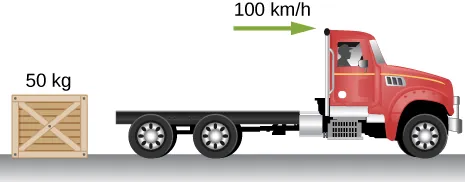114.

A 15-kg sled is pulled across a horizontal, snow-covered surface by a force applied to a rope at 30 degrees with the horizontal. The coefficient of kinetic friction between the sled and the snow is 0.20. (a) If the force is 33 N, what is the horizontal acceleration of the sled? (b) What must the force be in order to pull the sled at constant velocity?

115.

A 30.0-g ball at the end of a string is swung in a vertical circle with a radius of 25.0 cm. The tangential velocity is 200.0 cm/s. Find the tension in the string: (a) at the top of the circle, (b) at the bottom of the circle, and (c) at a distance of 12.5 cm from the center of the circle $(r=12.5cm).(r=12.5cm).$

116.

A particle of mass 0.50 kg starts moves through a circular path in the xy-plane with a position given by $r→(t)=(4.0cos3t)i^+(4.0sin3t)j^r→(t)=(4.0cos3t)i^+(4.0sin3t)j^$ where r is in meters and t is in seconds. (a) Find the velocity and acceleration vectors as functions of time. (b) Show that the acceleration vector always points toward the center of the circle (and thus represents centripetal acceleration). (c) Find the centripetal force vector as a function of time.

117.

A stunt cyclist rides on the interior of a cylinder 12 m in radius. The coefficient of static friction between the tires and the wall is 0.68. Find the value of the minimum speed for the cyclist to perform the stunt.

118.

When a body of mass 0.25 kg is attached to a vertical massless spring, it is extended 5.0 cm from its unstretched length of 4.0 cm. The body and spring are placed on a horizontal frictionless surface and rotated about the held end of the spring at 2.0 rev/s. How far is the spring stretched?

119.

A piece of bacon starts to slide down the pan when one side of a pan is raised up 5.0 cm. If the length of the pan from pivot to the raising point is 23.5 cm, what is the coefficient of static friction between the pan and the bacon?

120.

A plumb bob hangs from the roof of a railroad car. The car rounds a circular track of radius 300.0 m at a speed of 90.0 km/h. At what angle relative to the vertical does the plumb bob hang?

121.

An airplane flies at 120.0 m/s and banks at a $30°30°$ angle. If its mass is $2.50×103kg,2.50×103kg,$ (a) what is the magnitude of the lift force? (b) what is the radius of the turn?

122.

The position of a particle is given by $r→(t)=A(cosωti^+sinωtj^),r→(t)=A(cosωti^+sinωtj^),$ where $ωω$ is a constant. (a) Show that the particle moves in a circle of radius A. (b) Calculate $dr→/dtdr→/dt$ and then show that the speed of the particle is a constant $Aω.Aω.$ (c) Determine $d2r→/dt2d2r→/dt2$ and show that a is given by$ac=rω2.ac=rω2.$ (d) Calculate the centripetal force on the particle. [Hint: For (b) and (c), you will need to use $(d/dt)(cosωt)=−ωsinωt(d/dt)(cosωt)=−ωsinωt$ and $(d/dt)(sinωt)=ωcosωt.(d/dt)(sinωt)=ωcosωt.$

123.

Two blocks connected by a string are pulled across a horizontal surface by a force applied to one of the blocks, as shown below. The coefficient of kinetic friction between the blocks and the surface is 0.25. If each block has an acceleration of $2.0m/s22.0m/s2$ to the right, what is the magnitude F of the applied force?124.

As shown below, the coefficient of kinetic friction between the surface and the larger block is 0.20, and the coefficient of kinetic friction between the surface and the smaller block is 0.30. If $F=10NF=10N$ and $M=1.0kgM=1.0kg$, what is the tension in the connecting string?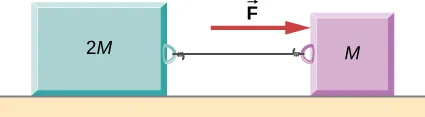125.

In the figure, the coefficient of kinetic friction between the surface and the blocks is $μk.μk.$ If $M=1.0kg,M=1.0kg,$ find an expression for the magnitude of the acceleration of either block (in terms of F, $μk,μk,$ and g).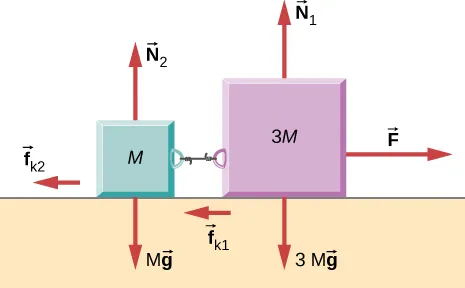126.

Two blocks are stacked as shown below, and rest on a frictionless surface. There is friction between the two blocks (coefficient of friction $μμ$). An external force is applied to the top block at an angle $θθ$ with the horizontal. What is the maximum force F that can be applied for the two blocks to move together?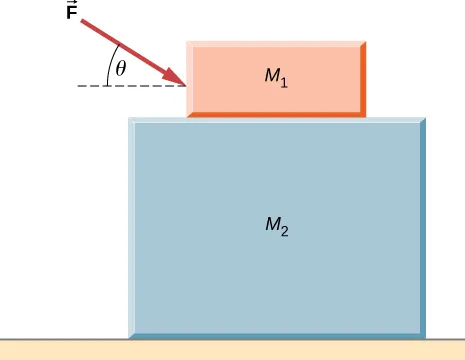127.

A box rests on the (horizontal) back of a truck. The coefficient of static friction between the box and the surface on which it rests is 0.24. What maximum distance can the truck travel (starting from rest and moving horizontally with constant acceleration) in 3.0 s without having the box slide?

128.

A double-incline plane is shown below. The coefficient of friction on the left surface is 0.30, and on the right surface 0.16. Calculate the acceleration of the system.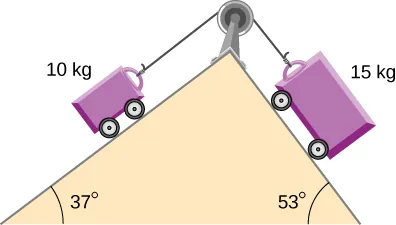Order a print copy

As an Amazon Associate we earn from qualifying purchases.